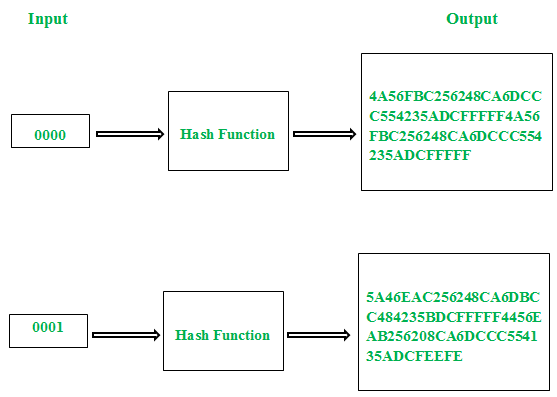# Avalanche Effect in Cryptography

In cryptography, the avalanche effect is a term associated with a specific behavior of mathematical functions used for encryption. Avalanche effect is considered as one of the desirable property of any encryption algorithm. A slight change in either the key or the plain-text should result in a significant change in the cipher-text. This property is termed as avalanche effect.

In simple words, it quantifies the effect on the cipher-text with respect to the small change made in plaint text or the key.Figure – Avalanche Effect

Even though the concept of avalanche effect was identified by “Shannon’s property of confusion”, the term was first mentioned by Horst Feistel. To implement a strong cipher or cryptographic hash function, this should be considered as one of the primary design objective.

In case of algorithm that uses hash value, even a small alteration in an input string should drastically change the hash value. In other words, flipping single bit in input string should at least flip half of the bits in the hash value.

A good encryption algorithm should always satisfy the following relation:

`Avalanche effect > 50% `

The effect ensures that an attacker cannot easily predict a plain-text through a statistical analysis. An encryption algorithm that doesn’t satisfies this property can favor an easy statistical analysis. That is, if the alteration in a single bit of the input results in change of only single bit of the desired output, then it’s easy to crack the encrypted text.

Examples:

Example-1: Avalanche effect in cryptography refers
(A) Large changes in cipher text when the keyword is changed minimally
(B) Large changes in cipher text when the plain text is changed
(C) Large impact of keyword change to length of the cipher text
(D) None of the above

Example-2: Avalanche effect in cryptography
(A) Is desirable property of cryptographic algorithm
(B) Is undesirable property of cryptographic algorithm
(C) Has no effect on encryption algorithm
(D) None of the above